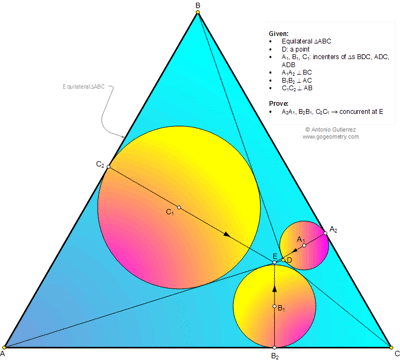## Monday, May 5, 2014

### Geometry Problem 1012: Equilateral Triangle, Incenters, Inscribed Circles, Perpendicular, Concurrent Lines

Level: Mathematics Education, High School, Honors Geometry, College.

Click the figure below to enlarge the figure of problem 1012.#### 1 comment:

1.To prove that A1A2, B1B2, C1C2 concurrent at a point E we need:
(C2B)^2 - (C2A)^2 + (B2A)^2 -(B2C)^2 + (A2C)^2 - (A2B)^2 = 0
Which is equivalent to C2B + B2A + A2C = C2A + B2C + A2B <=> C2B + B2A + A2C = 1.5a
We set AB = AC = BC = a
AD = x, BD = y; CD = z;
Since C2B = 0.5(y+a-x); B2A = 0.5(a+x-z); A2C = 0.5(a+z-y)
=> C2B + B2A + A2C = 1.5a
So: A1A2, B1B2, C1C2 concurrent at a point E